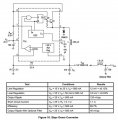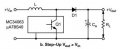# Voltage divider design questions

Thread Starter

#### kpgraci

Joined Jul 30, 2010
4
Hey all,
I want to convert a battery powered siphon pump powered by 2D cells (3v) to run off a 12v marine battery (12v 55Ah AGM). Some online research led me to the voltage divider circuit, and how to use ohm's law to figure out the resistance needed. But under load things change since the load adds a parrallel resistance, so at a minimum I need to figure out how the load will change the circuit. Now I can find the resistance of the motor easy enough by measuring its windings but I suspect there is more to it than that. Also there is the issue of current. I suppose I need to know how many amps the motor draws so the necessary circuit current can be determined, and that will tell me the resistance I need for r1 and r2, as well as their watt rating.
If you can't tell I have no circuit design training or experience but this seem like a doable project, so I guess I'll ask some questions now.
Is the votage divider the best circuit for this application?
Is so so did I misunderstand anything about the problem?
I can run the motor under load and measure it's current draw, but is the resistance it adds to the circuit just its winding resistance?
Did I forget to ask something I need to know?
And while I like projects and figuring things out if someone knows exactly how to build such a circuit I will gladly accept that as well!
Thanks!

#### dl324

Joined Mar 30, 2015
12,684
Is the votage divider the best circuit for this application?
Voltage dividers are only suitable for low currents. For larger currents, you waste a lot of power in the voltage divider because the divider current should be 10 times the load current.

What is the current draw of the motor? How close to 3V does the motor voltage need to be? Why didn't you consider a buck regulator?

#### crutschow

Joined Mar 14, 2008
26,786
Yes, a voltage divider is not appropriate for your application.
I can run the motor under load and measure it's current draw, but is the resistance it adds to the circuit just its winding resistance?
No.
The winding resistance basically determines the start current, which is typically much higher than the running current, so you need to measure the current under load.

If the current is no more a than a couple tenths of an amp, you could use an IC series linear regulator such as the common LM317.
If more, than a buck regulator, as dl324 suggested, will be more efficient (less waste heat to dissipate).

#### sparky 1

Joined Nov 3, 2018
490
years ago my car had a 12V to 6V voltage divider. it wasted a lot of power. Now we can use a buck converter, having other names:

A DC-DC step down converter module will convert 13.8 V DC to 3V DC like the LM2596 Step Down Module up to 92% efficient.
A 1.5V D cell is rated at minimum of 8000 mah (miliAmp hour) with typical circuit draw of 200mA
The step down converter would need to supply approx 3V @ 400 mA = 0.400 Amps
After adjusting the output for 3V the connections are straight forward 13.8V input and 3V output to the water pump.

Last edited:
•Kjeldgaard
Thread Starter

#### kpgraci

Joined Jul 30, 2010
4
Thread Starter

#### kpgraci

Joined Jul 30, 2010
4
So follow up question please - looking at the buck converter and trying to figure out how it works...

DC or rectified AC comes in, then a transistor converts that into a square wave (pulsed) then the flywheel circuit smooths it out, fine, how does that reduce the voltage? Is it the frequency of the square wave? the amplitude?

...and is seems some of these circuits can go the other way and boost DV volts, how would that work?

#### dl324

Joined Mar 30, 2015
12,684
DC or rectified AC comes in, then a transistor converts that into a square wave (pulsed) then the flywheel circuit smooths it out, fine, how does that reduce the voltage? Is it the frequency of the square wave? the amplitude?
A square wave is used to switch the pass transistor from saturation mode to cutoff. An LC filter is used to smooth the square waves. When the output rises to the desired output voltage, the comparator will prevent the transistor from switching. When the voltage drops below the target voltage, the comparator lets the transistor start switching again and the cycle repeats.#### Tonyr1084

Joined Sep 24, 2015
5,821
I want to convert a battery powered siphon pump powered by 2D cells (3v) to run off a 12v marine battery (12v 55Ah AGM).
That's a small pump.
If the current is no more a than a couple tenths of an amp, you could use an IC series linear regulator such as the common LM317.
If more, (then) a buck regulator, as dl324 suggested, will be more efficient (less waste heat to dissipate).
Agreed. LM317 or a Buck. Exactly how a buck works - it switches very fast and varies the pulse width. The end result is an average voltage out. Since you can adjust the buck to 3V it should serve nicely. The 317 route requires dropping 9V, which is a lot of wasted heat energy. A preferred method (IMHO) would be the buck. Regardless of how they work - they work. The 3V pump running on 2 D cells doesn't draw much power. But we still don't know how much power the pump draws. But a resistor divider isn't the way to go.

#### Tonyr1084

Joined Sep 24, 2015
5,821
some of these circuits can go the other way and boost DV volts, how would that work?
They invert and transform to a much higher voltage, then buck to the desired level. ONE CAVEAT: you don't get more power from a buck/boost converter. Power is the multiple of voltage times amperage. If you had 6V @ 2A (in a perfect world) you can convert that to 12V @ 1A. The power factor remains the same. 6x2 = 12x1. 6V at 2A is 12W, and 12V at 1A is 12W. In a Perfect world. There are losses and inefficiencies.

Going from 12V to 3V will still waste some power (watts) but no where near as much as the LM317 will lose.

#### dl324

Joined Mar 30, 2015
12,684
...and is seems some of these circuits can go the other way and boost DV volts, how would that work?
The energy stored in the inductor while the switching transistor is on is used to generate the higher voltage.

From ON Semiconductor AN920-D figure 7b:When Q1 is on, energy is stored in the inductor. When Q1 is turned off, the collapsing field in the inductor will generate a voltage across the inductor that is added to Vin and current will flow through D1.

•Tonyr1084

#### Tonyr1084

Joined Sep 24, 2015
5,821
Good explanation of how boost works.

#### crutschow

Joined Mar 14, 2008
26,786
An inductor will generate a voltage that will try to keep the current flowing when the transistor switch turns off, until the inductive energy is dissipated.
This voltage can be much higher than the voltage used to generate the inductor current (and inductive energy) during the time the transistor switch is on.

Similar threads